# Cramer's Rule Calculator

To solve the linear equations, input the values of variables in the Cramer’s rule calculator.

Give Us Feedback

This Cramer’s rule calculator can solve equations whether they are 2×2, 3×3, or 4×4 matrices.  You can see the step-by-step process of calculating the values of variables.

## What is Cramer’s rule?

Cramer’s rule is a method of finding values of variables of linear equations with matrices. It utilizes determinants in the formula to compute results.

Cramer's rule applies to the case where the coefficient determinant is nonzero.

## How to solve equations with Cramer's Rule - 2×2 matrix

Consider two linear equations as: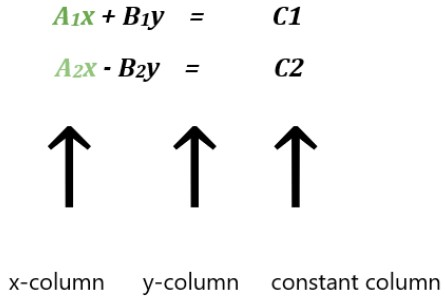Making matrices.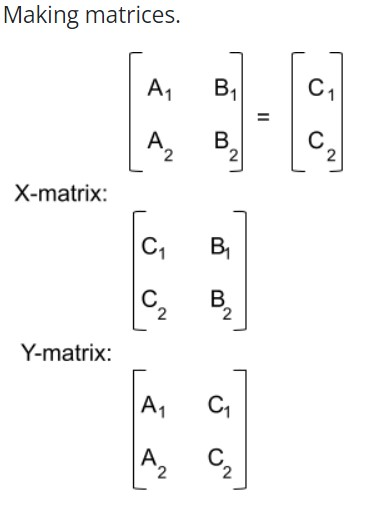Find determinants of all matrices.

i.e.   D, Dx, Dy

Value of x:

X = Dx/D

Value of y:

Y = Dy/D

Similarly, cramer's rule 4x4 and 3x3 can be determined but the linear equations will increase.

Example: For cramer's rule 2x2

Calculate the values of x1 and x2 of the given linear equations by using cramer rule method.

8x1 + 4x2 = 4

5x1 + 11x2 = 12

Solution

Step 1: First of all, write the linera equations in matrix form.

AX = B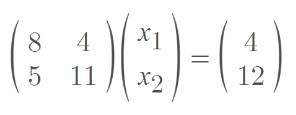Step 2: Now calculate the determinent of matrix A.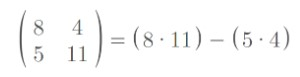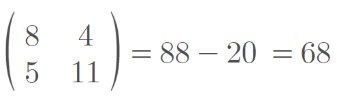Step 3: Now replce first column of matrix A by answer colum.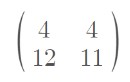Step 4: Find the determinant of the above matrix.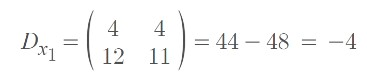Step 5: Now replce the second column of matrix A by the answer matrix.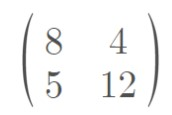Step 6: Find the determinant of the above matrix.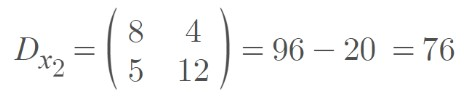Step 7: Now calculate the values of x1 & x2 by using formulas.

For x1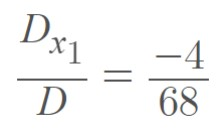x1 = -0.0588

For x2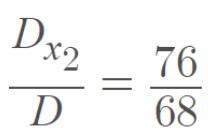x2 = 1.1176

### Math Tools